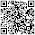Saturday, 17 December 2011

3d extrusion from 2d silhouette

For a while I have been looking for a way to import silhouettes into the 3d world.

I wanted to convert this image

I tried inkscape, by following the examples on the internet the examples worked fine. But combining this with importing a silhouette and edge detection only resulted in very nice cookie cutters vs solid objects.   I could not find any way to do what I wanted (but my skills with inkscape are limited)

I found no other program on the net that even came close, just lots of things making bumpmaps.

So I decided to try writing something myself to convert it to openscad code

V1. Just converted the black pixels to cubes eg "translate([61,118,0]) cube([1,1,1],center =false)"  This worked but in a not very usable way.
This just creates far to many polygons. I very quickly found out that openscad seems to have a limit of 9993 cubes.

V2. I added in some smarts to make the cubes as big as possable.
In doing this I discovered jpeg artifacts.  If you create a simple jpeg in gimp eg a black square on a while background and save it with a quality of less that 100%, it will add in additional colours! This confused the code no end as it was only expecting two colours. To get around this I converted the image to a black and white bitmap. This seemed to work very nicely.

I added in some random colours per cube so I could see what it was up to.This is what it looked like in openscad when compiled.
Compiling and rendering this took about an hour, still to many polygons.

This would not allow me to export though, it resulted in an error which was not self explanatory (sadly I didnt take note of it). Basically the error meant that you cant have two walls co-plainer, and all touching cubes are co-plainer.

I fixed this by adding a 0.0001mm to both x and y sizes of all cubes, resulting in cubes next to each other over lapping a fraction.

After this I was able to generate a .stl file. I further refined this with Meshlab

The result, I was able to print them!

This worked very well. So I though I would try something else...
Unfortunately everything else so far has produced scad files with far to many cubes.
Update, this turned out to be human error... this is what happens when you try things at 1am..   So I retract the above. All other silhouettes Ive tried so far do work!.

Here is the v2 code...  file names are hard coded and lots of features/debugging are turned on/off by adding or replacing the comments.

Hey its pre alpha, what do you expect?

There is at least one bug in this, when creating random colours the random number generator sometimes likes to create really small number written with exponents, openscad will error on these. I just edited the value to be 0 to get over it.

This is in python, I've never written any python before.

----------------------start of code----------------------
import Image, random
def findbox (x,y):
xlen = 0;
ylen = 0;
#       print '(x,y) = '+str(x)+' '+ str(y)
#       print 'current (x,y) '+ str(pix[x,y])
#       print 'current (x+1,y) '+ str(pix[x+1,y])
while (pix[x+xlen+1,y] != 0):
xlen = xlen + 1
#               print 'xlen = ['+str(xlen)+']'
#               print 'current (x+xlen+1) '+ str(pix[x+xlen+1,y]);
if x+xlen+2 >= im.size:
break

#       print 'start y current (x,y) '+ str(pix[x,y])
#       print 'current (x+xlen,y+ylen+1) '+ str(pix[x+xlen,y+ylen+1])
while (pix[x+xlen,y+ylen+1] != 0 and pix[x,y+ylen+1] != 0):
ylen = ylen + 1
#               print 'ylen = ['+str(ylen)+']'
#               print 'current (y+ylen+1) '+ str(pix[x+xlen,y+ylen+1]);

#               if (y+ylen)%((xlen+1)) == 0 :
#                       break
if y+ylen+2 >= im.size:
break

print 'color(['+str(random.random())+','+str(random.random())+','+str(random.random())+']) translate(['+str(x)+','+str(y)+',0]) cube(['+str(xlen+1+0.0001)+','+str(ylen+1+0.0001)+',2.5],center =false);'
#       print 'translate(['+str(x)+','+str(y)+',0]) cube(['+str(xlen+1+0.0001)+','+str(ylen+1+0.0001)+',10],center =false);'
im.paste((0),(x,y,x+xlen+1,y+ylen+1))

#       im.show()
return

im = Image.open("silhouette.bmp")
#im.show()
#print pix[0,0]
#print im.size

for y in xrange(im.size-1):
for x in xrange(im.size-1):
#print pix[x,y]
if pix[x,y] != 0:
findbox (x,y)

----------------------end of code-----------------------

Hope someone finds this useful

1 comment:

1.This version has issues with things like n shapes, it can go down both legs and fill in the empty space. I have a new version that checks the area really is filled in. Coming soon.